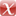# Contraction groups and the big cell for endomorphisms of Lie groups over local fields

H. Glöckner, ArXiv:2101.02981 (2021).

Preprint | English
Author
Abstract
Let $G$ be a Lie group over a totally disconnected local field and $\alpha$ be an analytic endomorphism of $G$. The contraction group of $\alpha$ ist the set of all $x\in G$ such that $\alpha^n(x)\to e$ as $n\to\infty$. Call sequence $(x_{-n})_{n\geq 0}$ in $G$ an $\alpha$-regressive trajectory for $x\in G$ if $\alpha(x_{-n})=x_{-n+1}$ for all $n\geq 1$ and $x_0=x$. The anti-contraction group of $\alpha$ is the set of all $x\in G$ admitting an $\alpha$-regressive trajectory $(x_{-n})_{n\geq 0}$ such that $x_{-n}\to e$ as $n\to\infty$. The Levi subgroup is the set of all $x\in G$ whose $\alpha$-orbit is relatively compact, and such that $x$ admits an $\alpha$-regressive trajectory $(x_{-n})_{n\geq 0}$ such that $\{x_{-n}\colon n\geq 0\}$ is relatively compact. The big cell associated to $\alpha$ is the set $\Omega$ of all all products $xyz$ with $x$ in the contraction group, $y$ in the Levi subgroup and $z$ in the anti-contraction group. Let $\pi$ be the mapping from the cartesian product of the contraction group, Levi subgroup and anti-contraction group to $\Omega$ which maps $(x,y,z)$ to $xyz$. We show: $\Omega$ is open in $G$ and $\pi$ is \'{e}tale for suitable immersed Lie subgroup structures on the three subgroups just mentioned. Moreover, we study group-theoretic properties of contraction groups and anti-contraction groups.
Publishing Year
Journal Title
arXiv:2101.02981
LibreCat-ID

### Cite this

Glöckner H. Contraction groups and the big cell for endomorphisms of Lie groups over  local fields. arXiv:210102981. Published online 2021.
Glöckner, H. (2021). Contraction groups and the big cell for endomorphisms of Lie groups over  local fields. In arXiv:2101.02981.
@article{Glöckner_2021, title={Contraction groups and the big cell for endomorphisms of Lie groups over  local fields}, journal={arXiv:2101.02981}, author={Glöckner, Helge}, year={2021} }
Glöckner, Helge. “Contraction Groups and the Big Cell for Endomorphisms of Lie Groups over  Local Fields.” ArXiv:2101.02981, 2021.
H. Glöckner, “Contraction groups and the big cell for endomorphisms of Lie groups over  local fields,” arXiv:2101.02981. 2021.
Glöckner, Helge. “Contraction Groups and the Big Cell for Endomorphisms of Lie Groups over  Local Fields.” ArXiv:2101.02981, 2021.

### Export

Marked Publications

Open Data LibreCat

### SourcesarXiv 2101.02981